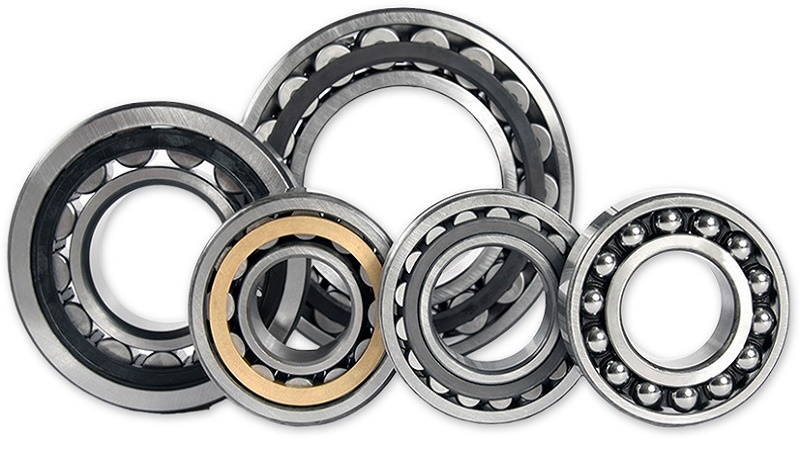Mechanics of Solids - CE6302

Online Study Material, Lecturing Notes, Assignment, Reference, Wiki and important questions and answersSTRESS, STRAIN AND DEFORMATION OF SOLIDS

=> Mechanics Of Solids: Rigid and deformable bodies
=> Mechanics Of Solids: General Concepts and Definitions
=> Mechanical properties of materials
=> True stress and true strain
=> Types Of Stresses
=> Deformation of simple bars under axial load Deformation of bodies
=> Deformation of compound bars under axial load
=> Mechanics Of Solids: Elastic constants
=> Important Question, Answer And Solved Problems: Civil - Mechanics Of Solids - Stress, Strain And Deformation Of Solids

=> Classification of Beams
=> Materials for Beam
=> Shear force and Bending Moment in beams
=> Stresses in beams
=> Effect of shape of beam section on stress induced
=> Important Question, Answer And Solved Problems: Civil - Transverse Loading On Beams And Stresses In Beam

TORSION

=> Torsion
=> Beam shear
=> Beams: Bars of Solid and hollow circular section
=> Beam: Stepped shaft ,Twist and torsion stiffness
=> Spring Deflection and Wahl's Factor
=> Important Question, Answer And Solved Problems: Civil - Mechanics Of Solids - Torsion

DEFLECTION OF BEAMS

=> Deflection Of Beams - Introduction
=> Evaluation of beam deflection and slope
=> Deflection Of Beams: Moment area method
=> Deflection Of Beams: Euler equation
=> Rankine formula for columns
=> Important Question, Answer And Solved Problems: Civil - Deflection Of Beams

THIN CYLINDERS and SPHERES AND THICK CYLINDERS

=> Triaxial Stress, Biaxial Stress, and Uniaxial Stress
=> Deformation in thin cylindrical and spherical shells
=> Stresses on inclined plane
=> Principal planes and stresses
=> Mohr's circle for biaxial stresses
=> Important Question, Answer And Solved Problems: Civil - Thin Cylinders, Spheres And Thick Cylinders